[top.htm]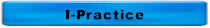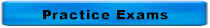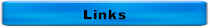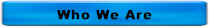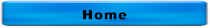More Math Problems & Charts

The controls for a pump are set for the pump to turn on when the pressure drops to 2 psi and to turn off when the pressure reaches 8 psi.  If this tank has a diameter of 50 feet, how many gallons will be stored between pumping cycles?

The controls for a pump are set for the pump to turn on when the pressure drops to 2 psi and to turn off when the pressure reaches 8 psi.  Calculate the following items:

1.  The depth of liquid when the pump turns on

2.  The depth of the liquid when the pump turns off

3.  The depth of the liquid that can be pumped

A centrifugal pump delivering 200 gpm against a total head of 40 feet produces _______ HP

A lot which measures 1200 feet by 1500 feet contains ______ acres

3 cubic yards contains _____ cubic feet

Calculate the pumping rate for a pump if the wet well drops 4 feet in 30 minutes. The wet well is 12 feet wide and 15 feet long.

If you dig a trench 500 feet long, 3 feet wide, and 8 feet deep, how many cubic yards of spoil will you remove?

What is the discharge pressure of your pump if you're pumping into a force main that is 40 feet above the pump and you need to maintain a pressure of 60 psi in the force main?

If the discharge pressure gauge reads 80 psi, what is the equivalent head in feet?

Calculate the flow for a 6-ft wide channel if the flow is 18 inches deep and the velocity is 2 fps,

6 mgd = _________ cfs

The temperature of your sample is 16oC.  What is this in oF?

If you thicken your solids from 2% to 5% and you typically pump 1000 gallons per day of 2%, how many gallons of 5% solids will you pump?

If you thicken your solids from 4% to 6% and you typically pump 12,000 gallons per day of 4%, how many gallons of 6% solids will you pump?

A lift station is equipped with three pumps, each rated at 175 gpm.   The wet well is 20 feet long, 10 feet wide, and 9 feet deep.  The average influent flow is 0.5 mgd.

• With no influent flow, one pump will empty the wet well in _______ minutes

• With no influent flow and one pump running, the water level in the wet well will drop ________ feet in 10 minutes

Given this lab data, calculate the TSS in mg/L

• Tare Weight  =  20.0012 g
• Final Dry Weight  =  20.0015 g
• Sample Volume  =  50 mL

Your sludge pump has one 12-inch piston with a 9-inch stroke.  It is rated at 50 RPM and is set to pump 2 minutes every 10 minutes.  The total solids concentration is 5%.  If the temperature of the raw sludge is 50oF and the digester is at 95oF, how many BTUs are required to warm the sludge?

Your sludge pump has one 12-inch piston with a 9-inch stroke.  It is rated at 50 RPM and is set to pump 2 minutes every 10 minutes.  The total solids concentration of the sludge is 5% and the volatile solids concentration is 70%.  Your digester typically produce 12 cubic feet of digester gas per pound of volatile solids fed to the digester and averages 650 BTU/cubic foot of digester gas, how many BTUs should be produced?

Your sludge pump has one 12-inch piston with a 9-inch stroke.  It is rated at 50 RPM and is set to pump 2 minutes every 10 minutes.  The total solids concentration of the sludge is 5% and the volatile solids concentration is 70%.  If your digester typically produce 12 cubic feet of digester gas per pound of volatile solids fed to the digester, how many cubic feet of digester gas should be produced?

Your sludge pump has one 12-inch piston with a 9-inch stroke.  It is rated at 50 RPM and is set to pump 2 minutes every 10 minutes.  If the total solids concentration is 5% and the volatile solids concentration is 70%, how many pounds of volatile solids are pumped daily?

Your sludge pump has one 12-inch piston with a 9-inch stroke.  It is rated at 50 RPM and is set to pump 2 minutes every 10 minutes.  If the total solids concentration is 5%, how many pounds of total solids are pumped daily?

Your sludge pump has one 12-inch piston with a 9-inch stroke.  It is rated at 50 RPM and is set to pump 2 minutes every 10 minutes How many gallons of sludge are pumped daily?

If a pump has one 12-inch piston with a 9-inch stroke, and is rated at 50 RPM, how many gpm will this pump deliver?

To determine the weight of a tank, you should:

A.  Calculate the volume and divide by 1,000,000

B.  Calculate the volume and multiply by 8.34

C.  Divide the depth by 2.31

D.  Divide the volume by the flow

mg/L x 8.34 x MGD is the formula for calculating

A. Chlorine demand

B. lbs

C. lbs/day

D. Volume of a cylindrical tank

Click on the thumbnail below for a chart of pump station math for cylindrical tanks and have some fun!!!

Click on the thumbnail below for a chart of pump station math for rectangular tanks and have some fun!!!

Click on the thumbnail below for a chart of pipe math and have some fun!!!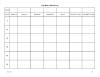Match 1 The units for pressure are A Divided by 2 Head is expressed in B 2.31 3 Velocity is measured in C 0.434 psi 4 Units of flow D psi 5 Pressure times _______ = Head E fps 6 Head __________ 2.31 = Pressure F 1440 7 20 psi = G 694 gpm 8 1 MGD = H cfs 9 A head loss of 7 feet = I 3 psi J Times K ft L 46.2 ft M Subtracted by

The formula for the volume of a rectangular tank is

A.  (Length)(Width)

B.  (0.785)(Diameter2)

C.  (Length)(Width)(Height)

D.  (0.785)(Diameter2)(Height)

The formula for the volume of a cylindrical tank is

A.  (Length)(Width)

B.  (0.785)(Diameter2)

C.  (Length)(Width)(Height)

D.  (0.785)(Diameter2)(Height)

Given a tank measures 50 feet long, 25 feet wide, and 15 ft deep, calculate:

• Surface area in ft2
• Cross-sectional area in square feet
• Volume in cf
• Capacity in gallons